> 文档中心 > 【数据结构与算法-- 树系列 -- 第一讲】哈夫曼树详解

# 【数据结构与算法-- 树系列 -- 第一讲】哈夫曼树详解

### 哈夫曼树详解

• 一、树
• 1.1、什么是树
• 1.2、树的定义
• 1.3、树的常用基本术语
• 1.4、树与非树
• 二、二叉树
• 2.1、什么是二叉树
• 三、Huffman 编码及实现
• 3.1、编码问题
• 3.2、使用二叉树解决编码问题
• 3.3、哈夫曼树的构造
• 四、哈夫曼树代码实现

# 一、树

## 1.1、什么是树

因为在客观世界里，有许多的事务，存在着细致的划分，比如下图：在我们中国，存储在各个省市的划分。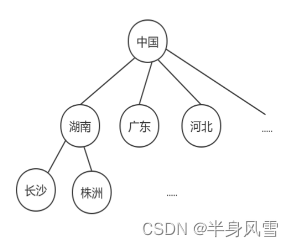我们可以看到，树这种结构在管理层次里面，它的管理效率更高。因为我们可以根据树的层次去更快的查找数据，比如：中国-湖南-广东-长沙等。树的层次管理具有更高的效率。

## 1.2、树的定义

• 树中有一个称为“`根(Root)`”的特殊结点。
• 其余结点可以分为若干个`互不相交的树`，称为原来结点的“子树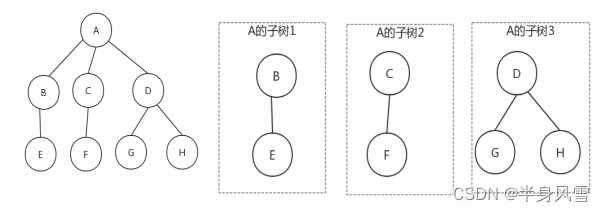## 1.3、树的常用基本术语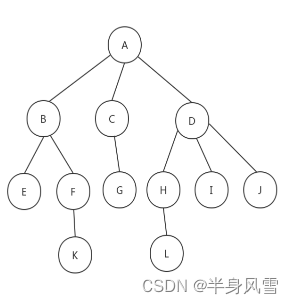• 结点的度：结点的子树个数。
• 树的度：树中所有结点中最大的度。
• 叶结点：度为0的结点。
• 父结点：所有子树的结点是其子树的根结点的父结点。
• 子结点：若A是B的父结点，B就是A的子结点。

## 1.4、树与非树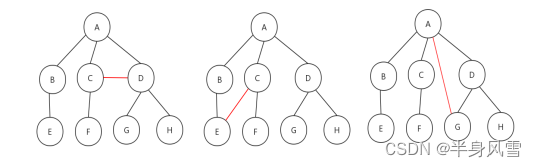• 子树是不相交的。
• 除了根结点之外，每个结点有且只有一个父节点
• 一个N个结点的树，只有N-1条边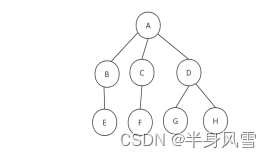# 二、二叉树

## 2.1、什么是二叉树

• 度为2的树（树中所有结点中最大的度）。
• 子树有左右之分。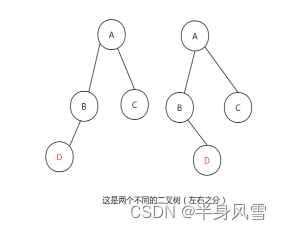# 三、Huffman 编码及实现

## 3.1、编码问题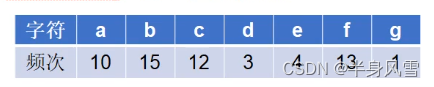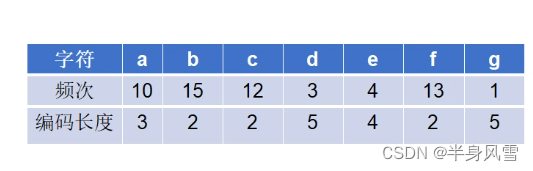1. 用等长ASCII编码：58 x 8 = 464位。
2. 用等长3位编码：58 x 3 = 174位。
3. 不等长编码：出现频次高的字符用的编码短些，出现频次低的编码长些。

`编码长度`：10 x 3 + 15 x 2 + 12 x 2 + 5 x 3 + 4 x 4 + 13 x 2 + 5 x 1 = 146位。

## 3.2、使用二叉树解决编码问题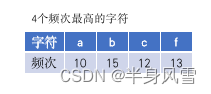b 编码 0
f 编码 1
c 编码 10
1 编码 11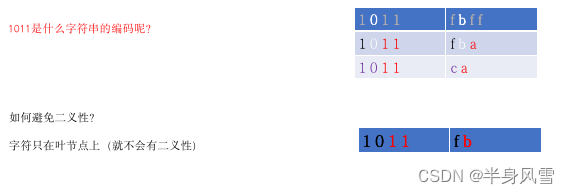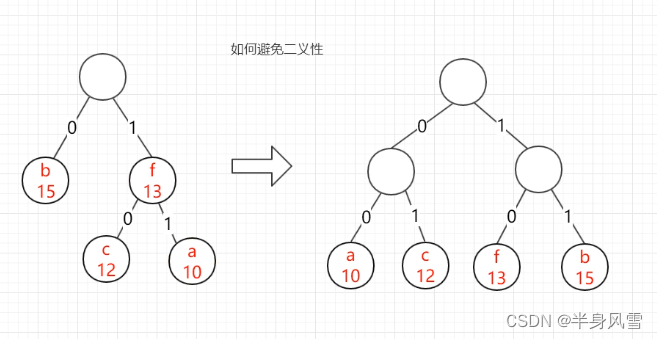# 四、哈夫曼树代码实现

``public class HuffmanTree {    //节点    public static class Node<E> { E data; //数据 int weight; //权重 Node leftChild; //左子节点 Node rightChild;//右子节点 public Node(E data, int weight) {     super();     this.data = data;     this.weight = weight; } public String toString() {     return "Node[" + weight + ",data=" + data + "]"; }    }    public static void main(String[] args) { List<Node> nodes = new ArrayList<Node>(); //把节点加入至list中 nodes.add(new Node("a", 10)); nodes.add(new Node("b", 15)); nodes.add(new Node("c", 12)); nodes.add(new Node("d", 3)); nodes.add(new Node("e", 4)); nodes.add(new Node("f", 13)); nodes.add(new Node("g", 1)); //进行哈夫曼树的构造 Node root = HuffmanTree.createTree(nodes); //打印哈夫曼树 printTree(root);    }    /**     * 构造哈夫曼树     *     * @param nodes     *     节点集合     * @return 构造出来的哈夫曼树的根节点     */    private static Node createTree(List<Node> nodes) { //如果节点node列表中海油2个和2个以上的节点 while(nodes.size()>1){     //什么是最小的，list表进行排序，增序的方式， 0,1，     sort(nodes);//排序方式是增序的     Node left = nodes.get(0);//权重最小的     Node right = nodes.get(1);//权重第二小的     //生成一个新的节点（父节点），父节点的权重为两个子节点的之和     Node parent = new Node(null,left.weight+right.weight);     //树的连接，让子节点与父节点进行连接     parent.leftChild = left;     parent.rightChild = right;     nodes.remove(0);//删除最小的     nodes.remove(0);//删除第二小的。     nodes.add(parent); } return nodes.get(0); //返回根节点    }    /**     * 冒泡排序，用于对节点进行排序（增序排序）     *     * @param nodes     */    public static void sort(List<Node> nodes) { if (nodes.size() <= 1)     return ; /*循环数组长度的次数*/ for (int i = 0; i < nodes.size(); i++){     /*从第0个元素开始，依次和后面的元素进行比较      * j < array.length - 1 - i表示第[array.length - 1 - i]      * 个元素已经冒泡到了合适的位置，无需进行比较，可以减少比较次数*/     for (int j = 0; j < nodes.size() - 1 - i; j++){  /*如果第j个节点比后面的第j+1节点权重大，交换两者的位置*/  if (nodes.get(j + 1).weight < nodes.get(j).weight) {      Node temp = nodes.get(j + 1);      nodes.set(j+1,nodes.get(j));      nodes.set(j,temp);  }     } } return ;    }    /*     * 递归打印哈夫曼树(先左子树，后右子树打印)     */    public static void printTree(Node root) { System.out.println(root.toString()); if(root.leftChild !=null){     System.out.print("left:");     printTree(root.leftChild); } if(root.rightChild !=null){     System.out.print("right:");     printTree(root.rightChild); }    }}``